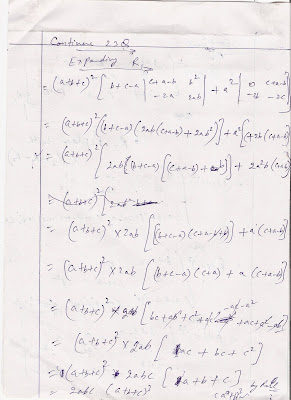## Friday, September 12, 2008

### Properties of Determinants

Here , I am giving you the full detail of properties of  determinant for your education purpose . The following properties are very helpful for commerce student to calculate value of determinant without using of expending procedure .

Just remember and use following properties of determinants :-

1. If we transpose of any matrix and then calculate the value of determinants, there is no difference between original value and transposed matrix’s determinant.
2. If all the elements of any one column or row of any matrix are zero, then the value of determinant must be zero.
3. If any two rows or column’s all value are same then the value of determinant must be zero.
4. If any two rows or column of any matrix are proportionate, then the value of determinant must be zero.
5. If we multiply any row or column with any scalar, its value of determinant will also multiply with same scalar.
6. If take any common factor from any column and write outside the matrix then the values of determinant never change.
7. If we calculate the value of any diagonal matrix then it always equal to the multiplication of its all main diagonals.
Following are the Scan images  of Example of  one Question which I have solved with the help of properties of determinant . I think this will be more helpful for you to undersand properties .Text is available under the Creative Commons Attribution-ShareAlike License; additional terms may apply. By using this site, you agree to our Privacy Policy. Mathematics Education® is a non-profit organization.